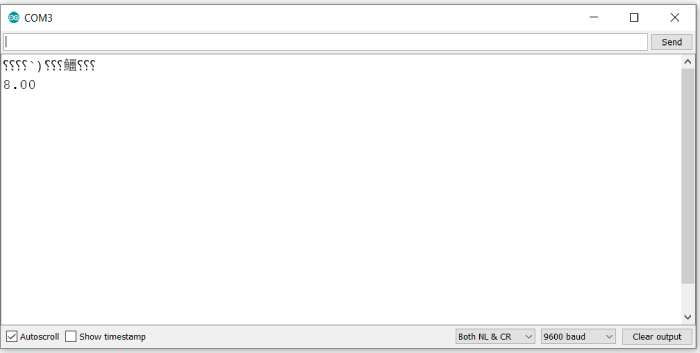# Exponential expressions in Arduino

The pow() function of Arduino can be used for evaluating exponential expressions. Any expression of the form ab can be expressed as pow(a,b). For example 23 becomes pow(2,3).

The type for both the base (a) and the exponent (b) is float. This function returns a double.

## Example

void setup() {
// put your setup code here, to run once:
Serial.begin(9600);
Serial.println();
float base = 2;
float exponent = 3;
Serial.println(pow(base,exponent));
}

void loop() {
// put your main code here, to run repeatedly:
}

## Output

The Serial Monitor Output is shown below −You are encouraged to try different values of base and exponent and check the working of this function.